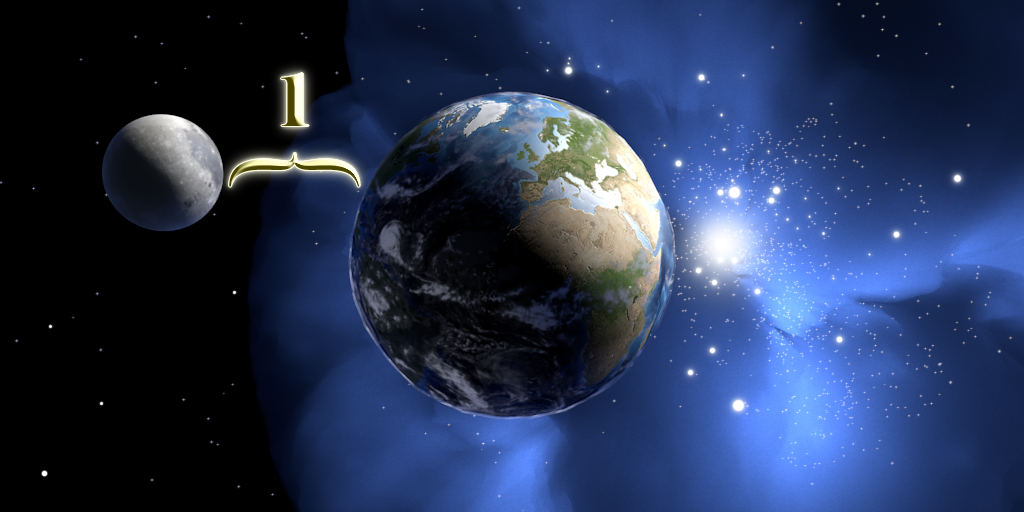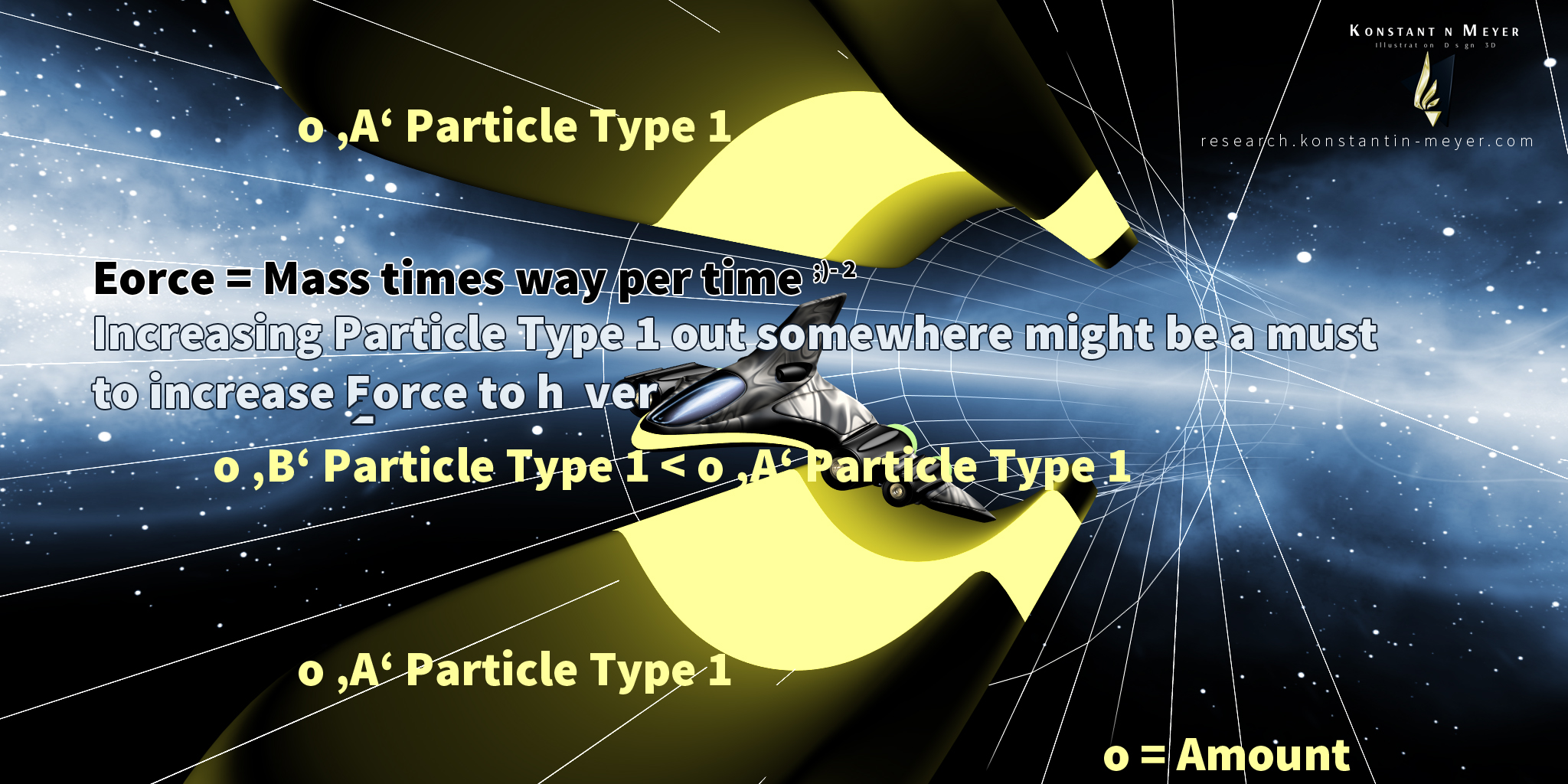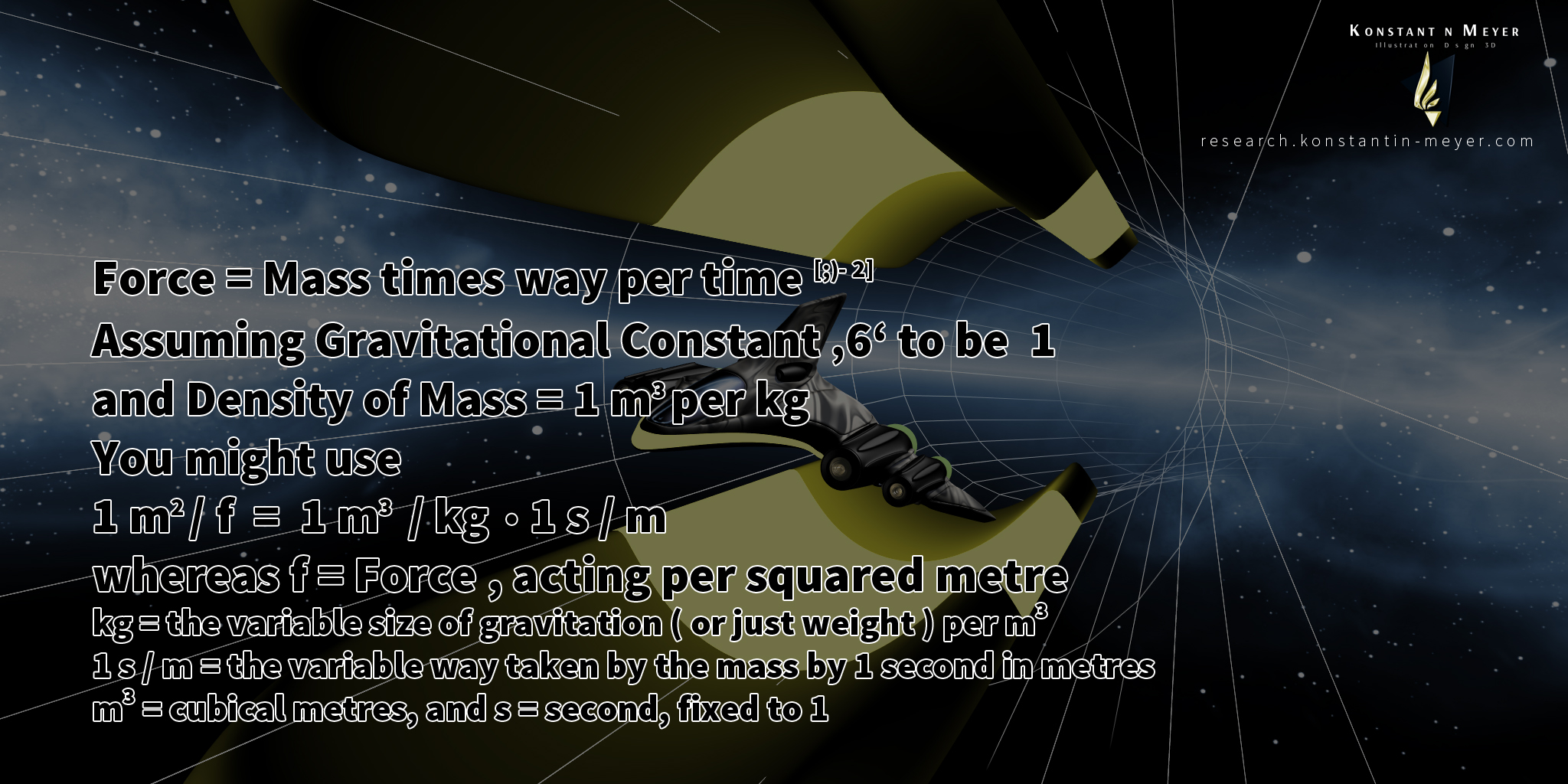Rese rch 1 . Solutions & Ideas .
Share this Site ' D N A ' if you like it .

' Assuming one Infinity, one Time is just a little grain of Sugar . '

Everything can be slighter by production without poisoning and new exploiting
with a strong recommendation to recycle and to clean from industrial toxics ,
and to take care for each other Living ! Stop ( y ) our war and helping on the way ?

8. Isolating Particle Types; Gravitational Force and its Constant '6' .

2021 :
The Gravitation Constant ' G ' has finally been determined !
Beside we are going to present the electromagnetic Constant ' Ge ' in the future – which has been worked out yet .
Simply get in contact with me for seeing the three ways to calculate ' G ' and the six possibilities to determine ' Ge ' !2016 - 2020 :
Gravitational Force and its Constant '6' .
And a trial to describe Force or Energy to be between the Minimum 0 'Zero' and a Maximum of 1 'One' .

Depending on relations; thinking about 1 cubic meter of air moving with 1000 meters per second
in relation to 1 cubic meter of wood moving with 1000 meters per second ;)-
which later went me to think about using 'mass density' and it seems to work fine :)

Call it just ' Density of force is density of mass times density of speed ' .

Based upon Isaac Newton and Albert Ein stein

Each relation has its Constant .

It should take 1 to calculating the gravitational Constant ' G '
because it might (? / !) lead to the logical inversion of 1 'One' ,
constantly nearing infinite close to 0 'Zero' - because time is missing.

Think Force of Moon per Force of Earth = roughly ' G '.
Think Force of Earth per Force of Sun = closer to ' G '
Think Force of Sun per Force of Milky Way Center = even closer to ' G '
Not only the Center of Milky Way is 'unsharp'
so that the Constant ' G ' will be approximate, too.
Continue these relations and you might go infinite close to 0 'Zero',
while 1 'One' K ( or kilogramm ) could be the Constant ' G ' beside
the Constant 1 'meter' and the Constant 1 'second' .

Still assuming differing ways ...
While 'logical inverted mass' is relative, too :)-

...

Gravitational Force attracts ( acts ) during time ,
so it should be possible to result ' Joules ' , too ,
by mass times speed squared ...

Acceleration due to gravity on Moon
should be ' exactly ' a hundred times less than on Earth ,
be the cause our Moon is about a hundred times lighter than our Earth :
1 kilogramm on Earth is half of 2 kilogramms on Earth ,
the same like Earth on 1 kilogramm or 2 times Earth on 1 kilogramm ,
may it to be gravitational force is getting less by its square alongside rising distance ...
Gravitational Force would be directly proportional to the planet ' s increasing mass value .

There are some ways to show that the gravitational Constant ' G ' should be 1 'One'.

Mass of Moon per two would be Speed times two ( and distance squared ) in order to still orbiting our Earth.
To be force the same :
f = (Mass 3x) /2 * (Speed 4x) * 2 = 12x ;
f = Mass 3 * Speed 4 = 12 ;
12 = 12x;
x = 1;

x/2 * y*2 = 1xy;
x*y = 1xy ;

And true, giving no ' just kidding :)- to the power of 2 '

be the cause

a Sun Light Particle needs exactly that impulse to leave gravitation of sun,

similar to air particles to be bound to gravitation of earth .See my site for more